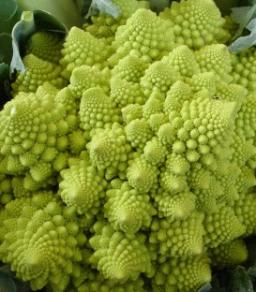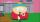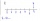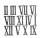# Two numbers

The sum of the two numbers is 1. Find both numbers if you know that half of the first is equal to one seventh of the second number.

a =  0.2222
b =  0.7778

### Step-by-step explanation:

a+b=1
a/2 = b/7

a+b = 1
7a-2b = 0

a = 2/9 ≈ 0.222222
b = 7/9 ≈ 0.777778

Our linear equations calculator calculates it.Did you find an error or inaccuracy? Feel free to write us. Thank you!Tips to related online calculators
Need help to calculate sum, simplify or multiply fractions? Try our fraction calculator.
Do you have a linear equation or system of equations and looking for its solution? Or do you have a quadratic equation?

## Related math problems and questions:

• Two numbersThe sum of two numbers is 1. Identify this two numbers if you know that the half of first is equal to the third of second number.
• QuizQ2The square of the first number is equal to three-fifths of the second number. Determine both numbers if you know that the second number is 5 times greater than the first, and neither of numbers is not equal to zero.
• Two numbersWe have two numbers. Their sum is 140. One-fifth of the first number is equal to half the second number. Determine those unknown numbers.
• Two unknown numbersOne number is 2 less than a second number. Twice the second number is 14 less than 5 times the first. Find the two numbers.
• The sum 8The sum of two numbers is 21. If three times the smaller numbers is two less than twice the larger number, find the two numbers.
• Three numbersFind three numbers so that the second number is 4 times greater than the first and the third is lower by 5 than the second number. Their sum is 67.
• Four-digit numbersFind four-digit numbers where all the digits are different. For numbers, the sum of the third and fourth digits is twice the sum of the first two digits, and the sum of the first and fourth digits is equal to the sum of the second and third digits. The di
• Ratio of two unknown numbersTwo numbers are given. Their sum is 30. We calculate one-sixth of a larger number and add to both numbers. So we get new numbers whose ratio is 5:7. Which two numbers were given?
• Difference of two numberThe difference between the two numbers is 20. They are positive integers greater than zero. The first number raised to one-half equals the second number. Determine the two numbers.
• Sum of three numbersThe sum of three numbers from which second number is 20% smaller than the first number and the third number is 25% smaller than the second number is 96. Determine this numbers.
• The largerThe larger of two numbers is nine more than four times the smaller number. The sum of the two numbers is fifty-nine. Find the two numbers.
• A number 4A number is made up of two digits. The sum of the digits is 11. if the digits are interchanged, the original number is increase by 9. Find the original number
• Four numbersThe first number is 50% second, the second number is 40% third, the third number is 20% of the fourth. The sum is 396. What are the numbers?
• The sum 16The sum of A and B is 36. The difference between A & B is 8. find the larger number of A.
• Sum and ratioThe sum and ratio of two numbers is equal to 10. Which numbers are they?
• The difference 2The difference between the two numbers is 25. The smaller number is 1/6th of the larger number. What is the value of the smaller number?
• Three shapes1/5 of a circle is shaded. The ratio of area if square to the sum of area of rectangle and that of the circle is 1:2. 60% of the square is shaded and 1/3 of the rectangle is shaded. What is the ratio of the area of circle to that of the rectangle?